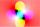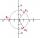# Pythagorean theorem + complex numbers - math problems

#### Number of problems found: 5

• ABS CNCalculate the absolute value of complex number -15-29i.
• Goniometric formDetermine the goniometric form of a complex number ?.
• StadiumA domed stadium is in the shape of spherical segment with a base radius of 150 m. The dome must contain a volume of 3500000 m³. Determine the height of the dome at its centre to the nearest tenth of a meter.
• Moivre 2Find the cube roots of 125(cos 288° + i sin 288°).
• BearingA plane flew 50 km on a bearing 63°20' and the flew on a bearing 153°20' for 140km. Find the distance between the starting point and the ending point.

We apologize, but in this category are not a lot of examples.
Do you have an interesting mathematical word problem that you can't solve it? Submit a math problem, and we can try to solve it.

We will send a solution to your e-mail address. Solved examples are also published here. Please enter the e-mail correctly and check whether you don't have a full mailbox.

Please do not submit problems from current active competitions such as Mathematical Olympiad, correspondence seminars etc...
Try our complex numbers calculator. Pythagorean theorem is the base for the right triangle calculator. Pythagorean theorem - math problems. Complex numbers - math problems.